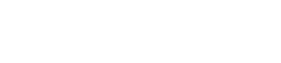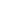NOW LIVEWork faster with Calculated Fields in Mode!Try it now## Mode Studio

The Collaborative Data Science Platform# SQL MIN/MAX

Starting here? This lesson is part of a full-length tutorial in using SQL for Data Analysis. Check out the beginning.

In this lesson we'll cover:

## The SQL MIN and MAX functions

`MIN` and `MAX` are SQL aggregation functions that return the lowest and highest values in a particular column.

They're similar to `COUNT` in that they can be used on non-numerical columns. Depending on the column type, `MIN` will return the lowest number, earliest date, or non-numerical value as close alphabetically to "A" as possible. As you might suspect, `MAX` does the opposite—it returns the highest number, the latest date, or the non-numerical value closest alphabetically to "Z."

For example, the following query selects the `MIN` and the `MAX` from the numerical `volume` column in the Apple stock prices dataset.

``````SELECT MIN(volume) AS min_volume,
MAX(volume) AS max_volume
FROM tutorial.aapl_historical_stock_price
``````

## Practice Problem

What was Apple's lowest stock price (at the time of this data collection)?

Try it out See the answer

## Practice Problem

What was the highest single-day increase in Apple's share value?

Try it out See the answer

Next Lesson

SQL AVG

Get the latest.